# Positions of GPS Satellites in 3D

2224
8
06-21-2021 01:28 PM
Labels (1)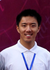byEsri Contributor
5 8 2,224

# Positions of GPS Satellites in 3D

TL;DR: See the positions of GPS satellites in the sky in real-time with ArcGIS Runtime.

Get the link to the source code at the bottom of this article.

## Abstract

• Many handheld GNSS/GPS receivers support tracking satellite positions in 2D
• Satellite positions are typically given by `\$GPGSV` sentences in NMEA specs, in the format of elevation and azimuth angles
• It is possible to calculate the approximate satellite positions in the sky, given the following parameters
• GPS satellite orbit altitude
• Elevation angle
• Azimuth angle
• No existing tool provides the functionality to display the GPS satellite position in real time, in 3D
• A concise way to calculate the satellite positions is proposed, using Swift and a bit of trigonometry
• Using the iOS app built with ArcGIS Runtime SDK, one can connect to a GPS receiver and see the satellite positions in 3D

## Introduction

When NMEA support was introduced in ArcGIS Runtime 100.10, it opens up lots of possibilities. Via NMEA sentences, these high-accuracy GNSS receivers provide not only highly accurate location data, but also a plethora of additional information, such as accuracy, heading, and GPS satellites in view.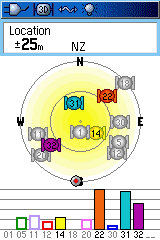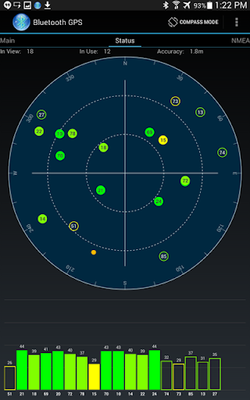Many handheld GPS receivers more or less support viewing the satellite information. On some devices, they show up as a series of signal bars; on other devices, two concentric circles are used to depict the earth and the orbit, and the satellites are plotted on that graph. These graphics are OK, but neither intuitive nor informative.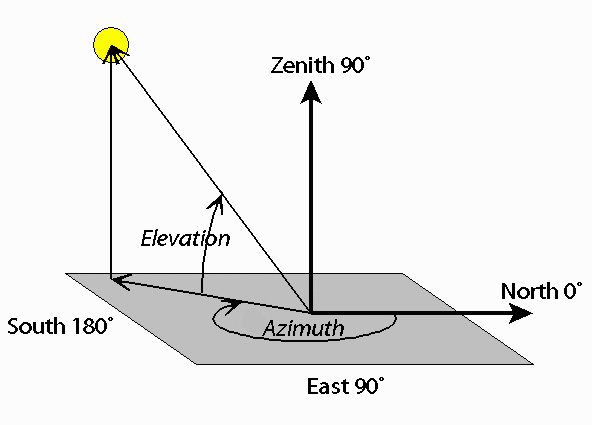The positions of satellites are represented by elevation and azimuth angles in `\$GPGSV` sentences in the NMEA specs. What are these? In short, azimuth tells us what direction to face, and elevation tells us how high up in the sky to look. Think of a person standing on the ground, holding both a GPS receiver and a compass. Turning around from the north and raising the head, one can eye into the approximate direction of the satellite in the sky with these 2 angles. However, when it comes to mapping software, things get a bit trickier.

How to represent azimuth and elevation on a map, or a 3D globe? Well, we know the current device location, or the lat-lon coordinates on the surface of the earth. We also know where we should look at with the 2 angles. The problem is - the map's spatial reference is somehow represented in 2D. Because the earth is a globe, normally we don't take the radius of the earth into account, as it is always 6371 km. In order to convert our lat-lon coordinates into the 3D space, some trigonometry has to be done.

## Methods

### Gather Information

At first, I tried to search online for existing algorithm to convert azimuth-elevation coordinates into the common sense lat-lon coordinates. To my surprise, no success. The `GeometryEngine` APIs in ArcGIS Runtime do provide a set of useful methods for geodetic calculation, but they are limited to the surface of the earth. It seems I have to come up with the trigonometry myself.

### Analyze the Problem

The first thought that came to mind is to convert all the coordinates into a 3D Cartesian coordinate system. The problem is - it is very hard and error-prone to correctly represent all the angles in xyz's. Converting the lat-lon is not too bad - just a few rectangular to polar conversions. But what about the azimuth angle - how to represent it in Cartesian? It led me towards thinking of vector operations and matrices.

Actually, the problem can be expressed succinctly in a spherical coordinate system. The resulting coordinates of the satellite are the summation of 2 vectors: the one from the center of the earth to the current location, and the other one from the current location to the satellite in its orbit, represented by azimuth and elevation angles.

It is still quite hard to visualize the spatial relationships of these vectors in the spherical coordinates though. Having drawn quite a few tangent planes on paper, I decided to draw the whole thing in 3D to make it clearer.

### 3D Geometry, Matrix and RotationsAt this point, I found a GitHub repo that contains some helpful code from Michael Fogleman, an engineer at Formlabs. Despite his calculation being not correct, the code in the repo pointed me to the right direction. (Also, the visuals on his personal webpage were pretty sleek!) It convinced me that the calculation can be done correctly, and I can create some really nice-looking stuff with the power of ArcGIS Runtime.

Two more major discoveries made this calculation feasible.

• First, SIMD instructions

In Michael's implementation, he does the matrix multiplication in Python by hand. Even if it is possible to replicate in Swift, it is pretty cumbersome to include a whole bunch of tedious math in such an elegant app. Luckily, Swift has a handy `simd` library. It supports up to 4×4 elements in a matrix, which is a perfect fit for our next purpose: quaternions.

• Second, Quaternions

Honestly speaking, I've never heard of this term in English before, so even though I thought about matrix in the first place, I didn't know where to start. When I saw its wiki page, my physics classes memory struck me. It's a very common practice to represent the rotation of a vector with a matrix, and that's what it's called in English!

The lat-lon coordinates can be effectively represented by quaternions. Consider a unit vector of the earth, with initial point at the center of the earth and terminal point at 0° East. By rotating this vector along the y-vector by latitude degrees, and z-vector by longitude degrees, we will get it point at the lat-lon position. More will be covered in the following section.

### The Quest to Find “The 3rd Axis”

So far, I've figured out how to use quaternions to facilitate the calculation. From the unit vector to the lat-lon vector, we did 2 rotations using y-vector and z-vector as the first and second axes. To get our resulting satellite vector though, we need a third axis to incorporate azimuth and elevation angles.

This is the trickiest part of math for this problem. To get there, we'll need to rotate a vector according to the elevation angle. That vector itself needs to be rotated from another vector by the azimuth angle. And I'm calling this vector the 3rd axis.

It’s hard to describe it in words, so I drew it all on GeoGebra. Toggle the graphics on the left pane to see their spatial relationships.

With 3 axes, 4 quaternions and all the mysterious steps, how to ensure the result is correct? Luckily again, with the visuals created on GeoGebra, I can get the azimuth and elevation values reversely, by specifying the other parameters, namely latitude, longitude, earth radius and orbit radius. So, if my calculation is correct, the resulting satellite coordinates should be the same as on GeoGebra.

## Results

### Validate the Algorithm

Attached is the preliminary version of the algorithm for calculating satellite lat-lon coordinates.

``````/// The function to get satellite position.
/// - Parameters:
///   - longitude: The longitude of GPS receiver location in degrees.
///   - latitude: The latitude of GPS receiver location in degrees.
///   - elevation: The elevation angle in degrees.
///   - azimuth: The azimuth angle in degrees.
///   - satelliteAltitude: The satellite altitude in kilometers.
///   - earthRadius: The earth radius in kilometers.
func snippet(longitude: Double, latitude: Double, elevation: Double, azimuth: Double, satelliteAltitude: Double = 20200, earthRadius: Double = 6371) {
// 1. Basic trigonometry
let r1 = earthRadius
let r2 = r1 + satelliteAltitude
let elevationAngle = radians(from: elevation) + .pi / 2
let inscribedAngle = asin(r1 * sin(elevationAngle) / r2)  // Law of Sines
let centralAngle = .pi - elevationAngle - inscribedAngle  // Internal angles sum = pi

// 2. Magic of quaternions
var latLonAxis: simd_double3

let xVector = simd_double3(1, 0, 0)
let yVector = simd_double3(0, 1, 0)
let zVector = simd_double3(0, 0, 1)

// Rotate negative latitude angle along y axis.
let quaternionLatitude = simd_quatd(
axis: yVector
)
latLonAxis = quaternionLatitude.act(xVector)
// Rotate longitude angle along z axis.
let quaternionLongitude = simd_quatd(
axis: zVector
)
latLonAxis = quaternionLongitude.act(latLonAxis)

// The 3rd axis is on the earth's tangent plane of device location.
// Note: the division by zero issue will be discussed in the addendum.
var thirdAxis = simd_normalize(simd_double3(0, 0, 1 / latLonAxis.z) - latLonAxis)
// Rotate azimuth angle. Since azimuth is defined as a clockwise angle,
// it needs to be negative. Also add pi to the result so that it serves
// as the axis for central angle rotation.
let quaternionAzimuth = simd_quatd(
angle: 1.5 * .pi - radians(from: azimuth),
axis: latLonAxis
)
thirdAxis = quaternionAzimuth.act(thirdAxis)
let quaternionElevation = simd_quatd(
angle: -centralAngle,
axis: thirdAxis
)
let resultVector = quaternionElevation.act(latLonAxis)

// 3. Print result
let resultLatitude = degrees(from: .pi / 2 - acos(resultVector.z))
let resultLongitude = degrees(from: atan2(resultVector.y, resultVector.x))
print(resultLongitude, resultLatitude)
}``````

It can be split into 3 parts - basic trigonometry, quaternion rotation and coordinate system conversion.First, as described above, we need to do some basic trigonometry on the "elevation plane", i.e. the plane where the elevation angle exists. Using Law of Sines, we can get the value of the central angle, assuming both the earth radius and the satellite orbital distance are constants.

Second, do the rotations.

• Rotate the x unit vector (0° East) to get the `latLonAxis` vector, which starts at the center of the earth, and ends at our longitude and latitude on the earth surface (lat-lon position).
• In order to find the axis for the elevation angle rotation, we need to first create the `thirdAxis` - which is a vector that runs perpendicular to the rotated azimuth vector. The azimuth vector itself, is a line on a tangent plane of the earth (which the point of tangency is the lat-lon position) that, by definition, starts at the lat-lon position and ends at 0° North, which intersects with the z axis.
• Ensure the sign of these angular values match the direction of rotation.

Finally, convert the Cartesian coordinates back to the (geographic) spherical coordinates.

There are a few possible ways to test the algorithm's correctness. The most accurate and handy way is to run the values from GeoGebra. As they match perfectly, I'm pretty confident the calculation is correct. One can also run some ad-hoc testing with the real data from a GPS receiver. For example, we should expect all the satellites-in-view to be above rather than below the horizon; If a satellite has a very small elevation angle and the result does show it just above the horizon and vice versa, then it's very likely that we've done it right!

### Create the Visuals with ArcGIS Runtime

With the powerful scene view APIs in ArcGIS Runtime, we can display spatial graphics in 3D with ease. One can even draw the satellites with 3D models, but here I just use red and gray dots. Combining with other APIs, within a few lines of code, we can draw the current lat-lon position as well as all the satellites in the sky, in real time.

### Brush Up Coding Style

Before I finally wrap up the project, some coding style tweaks need to be done.

• Bluetooth connection

Apple's Bluetooth accessory picker API isn't very easy to use. To make it easier to connect to a Bluetooth GPS receiver, I need to wrap them in helper methods.

Luckily, no more hassle after the device is connected - from 100.10, ArcGIS Runtime provides really handy APIs to setup the NMEA communication between the iOS device and the GPS receiver. A few lines of code and we are all set! The incoming NMEA messages will be parsed into location and satellite info objects automatically.

• Swift `Measurement`

To get rid of the magic numbers and unit conversions, `Measurement` objects in the `Foundation` framework come into play. With explicitly defined units, we can leverage the unit conversion methods (such as radians to degrees), so that people would be less likely to get confused.

## Conclusion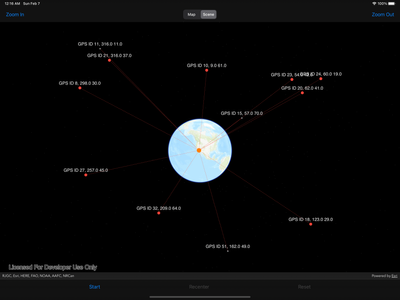Whoa! At first, I thought it was a simple problem, but it results in this long-winded article that tries to explain every aspect of the process. The optimized core algorithm is short and sweet - with only 2 quaternions, it would be very unlikely to have an even simpler way to get these satellite coordinates.

The resulting graphics are elegant and beautiful. When I first revealed the screen recordings, people are responding with starstruck emojis 🤩. To be fair, who won't be curious to see them in a perceivable graphical representation, rather than the abstruse numerics? Personally, I love the fact that these satellites are just there, orbiting above our heads and working tirelessly to connect everyone, everywhere.

And best of all - one can get all for free with great ease! No account or subscription required to try it out. Download the source code, and SwiftPM will help you setup ArcGIS Runtime automatically.

## References

1. GeoGebra 3D plot: https://www.geogebra.org/calculator/gzpa7gqe
2. `GPGSV` sentence definition: http://aprs.gids.nl/nmea/#gsv
3. GPS satellite orbit altitude: https://www.gps.gov/systems/gps/space
4. .NET sample for GPS satellites in view in 2D: https://github.com/Esri/arcgis-runtime-demos-dotnet/tree/main/src/ExternalNmeaGPS
5. What are the "azimuth and elevation" of a satellite? https://www.celestis.com/resources/faq/what-are-the-azimuth-and-elevation-of-a-satellite/
6. GitHub repo of Michael Fogleman: https://github.com/fogleman/GPS
7. Michael's GPS satellite visuals: http://www.michaelfogleman.com/gps/
8. GeoGebra 3D plot of the problem: https://www.geogebra.org/calculator/gzpa7gqe
9. Apple SIMD library: https://developer.apple.com/documentation/accelerate/simd
10. Working with Matrices: https://developer.apple.com/documentation/accelerate/working_with_matrices
11. Working with Quaternions: https://developer.apple.com/documentation/accelerate/working_with_quaternions
12. Wiki for rotation matrix: https://en.wikipedia.org/wiki/Rotation_matrix#Rotation_matrix_from_axis_and_angle
13. Wiki for coordinate system conversion: https://en.wikipedia.org/wiki/Spherical_coordinate_system#Cartesian_coordinates
14. Michael's quaternion implementation in Python: https://github.com/fogleman/pg/blob/master/pg/matrix.py#L196

## Acknowledgments

1. The Stack Exchange questions that directed me to the right path: https://gis.stackexchange.com/questions/274941 and https://gis.stackexchange.com/questions/220210
2. GeoGebra, the online free 3D graphic calculator
3. Michael Fogleman's Python code helped me to better clarify the problem
4. Various webpages that helped me understand NMEA specs and how GPS satellites work

## Update on 2021-07-14

After the article being posted, I've received bunch of useful feedback and compliments. Among them, 2 specific requests drew my interest. So here is a small update to the original article.

P.S. I've also updated the references with a few coordinate system conversion wiki pages added.

• What happens when the latitude is 0°?

This is a case that I haven't taken into consideration in the earlier implementation. Thanks to Anthony's comment below, I found there is the division by zero issue in my code.

It happens on the line where I tried to get the vector between ground location and 0° North. This vector should lie in the tangent plane of the ground lat-lon position. However, if the ground location is on the equator (say you are in Quito, Ecuador), the tangent plane is parallel to the z axis, which introduced some confusion. Having searched online, I cannot fine a definitive answer on what is the azimuth angle for a location on the equator. It seems that GPS receiver manufacturers handle this issue differently.

Anthony himself proposed a workaround to add a small deviation when latitude equals zero, and I think it is valid given that my previous calculations aren't highly accurate.

The other way is to handle the division by zero specifically. As we approach the equator from the Northern Hemisphere, the limit of the third axis equals the z unit vector. If the azimuth angle reported by the GPS receiver is as expected, the result should be correct. But it can also be totally wrong if the azimuth angle turns into a default fallback value or something.

``````var thirdAxis: simd_double3
if latLonAxis.z == 0 {
thirdAxis = simd_double3(0, 0, 1)
} else {
thirdAxis = simd_normalize(simd_double3(0, 0, 1 / latLonAxis.z) - latLonAxis)
}``````

After all, I cannot be sure how would a GPS receiver behave on the equator, so I'll probably have to actually visit a city on the magical line to test it out! 😉 (Quito added to bucket list)

• How would AR experience play out of this demo?

It's pretty straightforward to think of plotting the satellites in a AR scene, so that when we point the iPhone to the sky, we can see the satellites fly above our head. That would be pretty amazing and educational if people want to learn more about GPS. Therefore, I added a third AR mode to the demo app!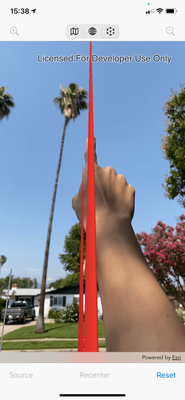Due to technical limitations and the parallax effect, I couldn't effectively plot the satellites in their real positions, which are around 20,200 km above our head. Instead, I added 3D leader lines between the ground location and the satellites. You can see through your AR camera, and use your finger to point towards the approximate direction of a satellite. I have to admit - it isn't as intuitive as to draw the satellites in the sky. But it is still a fun experience, and would be helpful if you are trying to observe the satellites with a telescope. You can use this app to do a coarse adjustment of its direction!

Tags (5)